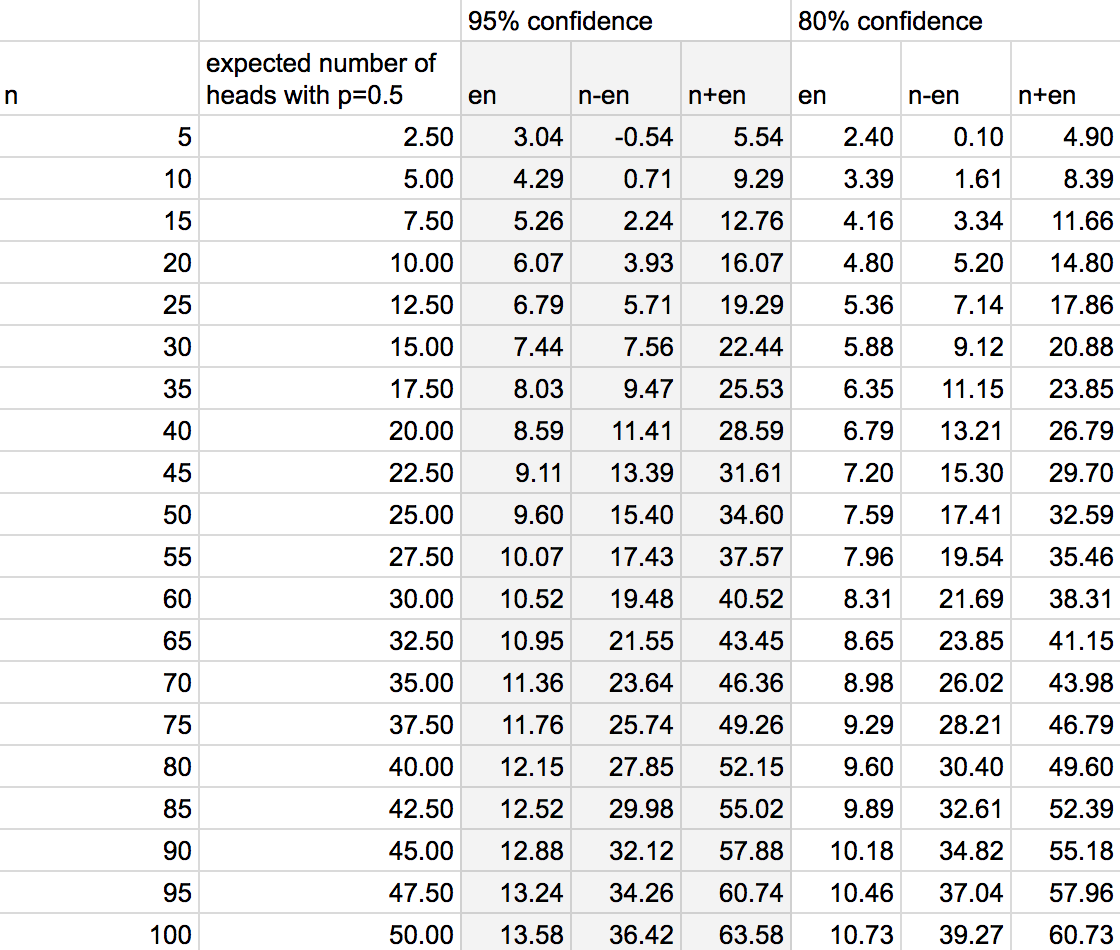# KarlRosaen

Creating a confidence interval using Hoeffding's inequality

Wrapping up the reading on inequalities (Wasserman's All of Statistics Chapter 4), the book shows how Hoeffding's inequality can be used to construct a confidence interval on the parameter $p$ for a binomial random variable.

Starting with Hoeffding's inequality (as applied to a series of samples of a Bernoulli, e.g a series of coin flips):

$$P(|\bar{X}_n - p| > \epsilon) \leq 2e^{-2n\epsilon^2}$$

we previously showed how this can be used to place bounds on the true error rate of an estimator given the observed error rate of a classifier on a training set. Switching examples to a series of coin flips, the analogous question is: if we flip a coin with true weight $p$ 100 times, what is the probability that the observed number of heads is within 20% of p?

What if, instead, we'd like to place +/- bounds on the number of flips to expect given a particular weight? We ask, if I flip the coin 100 times, how many flips on either side of $p*100$ will trap the observed number of flips, say, 90% of the time? Another way of saying this is, what is the 90% confidence interval on coin flips?

Given a particular probability bound $\alpha$:

$$2e^{-2n\epsilon^2} = \alpha$$

we can solve for $\epsilon$. We'll call this $\epsilon_n$:

$$\epsilon_n = \sqrt{\frac{1}{2n}log(\frac{2}{\alpha})}$$

leaving us with

$$P(|\bar{X}_n - p| > \epsilon_n) \leq \alpha$$

now if we consider the random interval $C = (\bar{X}_n - \alpha, \bar{X}_n + \epsilon_n)$ the probability that the number of heads falls outside of the interval is $P(p \notin C) = P(|\bar{X}_n - p| > \epsilon_n) \leq \alpha$. This means $P(p \in C) \geq 1 - \alpha$; the random interval $C$ traps the true parameter $p$ with probability $1-\alpha$. We call $C$ a $1-\alpha$ confidence interval.

To make this a bit more concrete, I created a gsheet that explores the confidence intervals around coin flip experiments. I set $p$ to 0.5 for the sake of example, but the interval is independent of $p$.### Recovering PGM course materials

I was annoyed to find that the materials for the coursera course on probabilistic graphical models have been removed pending a relaunch of the course on some new platform. Thankfully I found a torrent of the materials. To save you the trouble of navigating shady blogs to find the torrent file, I'll host it here too for a bit.